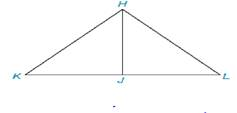Chapter 3.2, Problem 16E### Elementary Geometry for College St...

6th Edition
Daniel C. Alexander + 1 other
ISBN: 9781285195698

#### Solutions

Chapter
Section### Elementary Geometry for College St...

6th Edition
Daniel C. Alexander + 1 other
ISBN: 9781285195698
Textbook Problem
35 views

# Given: H J → bisects ∠ K H L H J ¯ ⊥ K L ¯ In Exercise 15, you cam delete Step 5 and 6 and still prove that “ ∠ K ≅ ∠ L ”. What reason would you use to establish that ∠ K ≅ ∠ L in the shorter proof.Prove: ∠ K ≅ ∠ L PROOF Statements Reasons 1. ? 1. Given 2. ∠ J H K ≅ ∠ J H L 2. ? 3. H J ¯ ⊥ K L ¯ 3. ? 4. ∠ H J K ≅ ∠ H J L 4. ? 5. ? 5. Identity 6. ? 6. ASA 7. ∠ K ≅ ∠ L 7. ?

To determine

To prove:

The given statement.

Explanation

Given:

The following figure shows the given diagram.

Given that,

HJ bisects KHL and,

HJ¯KL¯

If two angles of a triangle are congruent, then the third angle will also be congruent.

Approach:

The completed proof is shown in the following table.

 PROOF Statements Reasons 1. HJ→ bisects ∠KHL 1. Given 2. ∠JHK≅∠JHL 2. HJ→ bisects ∠KHL in two equal parts. 3. HJ¯⊥KL¯ 3. Given 4. ∠HJK≅∠HJL 4. HJ¯⊥KL¯ and KJ¯≅JL¯ 5

### Still sussing out bartleby?

Check out a sample textbook solution.

See a sample solution

#### The Solution to Your Study Problems

Bartleby provides explanations to thousands of textbook problems written by our experts, many with advanced degrees!

Get Started